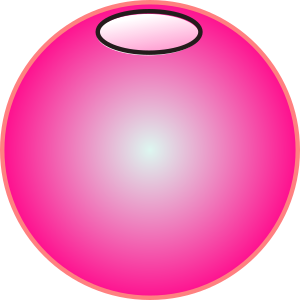## Wednesday 1st March 2017

Before getting into the main theme of this newsletter let’s begin with this month’s puzzle.

A 10cm long cylindrical hole is drilled straight through the centre of a solid sphere. What is the volume of the remaining part of the sphere? The answer is at the end of this newsletter.A good mathematics exercise is structured to lead the learner through from simple, confidence-building questions to harder questions that deepen understanding. In addition to this an exercise should provide the repetitive practice needed to fuse the method into long term memory.

To prevent this repetitive practice becoming boring the exercise could be presented in the form of a game so that the same objectives are achieved in a more fun-filled, exiting manner. Here are seven Transum games that work well with learners of a wide range of ages.

• Pairs Games for remembering connections whether it be parts of a circle and their names or common fractions and their decimal equivalents.
• Digital Darts is an online game for one or two players requiring skill, strategy and lots of mental arithmetic.
• Rounding Snap is a fast and furious game. If the last card put down equals the previous card to the nearest whole number then all players race to shout SNAP!
• Connect 4 Factors a game for one or two players. The winner is the first to line up four numbers with a common factor.
• Choose Your Average is a game for two players. You should know how to find the mean, median and range of a set of numbers before starting this game.
• Two Dice Bingo is a whole class game. Use it to provide a purpose for drawing up a possibility space.
• Hi-Low Predictions A version of the Play Your Cards Right TV show. Calculate the probabilities of cards being higher or lower.

And two brand new games this month both provide practice related to number sequences:

Watsadoo requires quick thinking. Players need to quickly identify the falling numbers as odd, even, square, cube, triangular or prime. At the time of writing no one has successfully complete a level greater than two.

The Square Pairs Game is for two players who take turns to select two numbers that add up to a square number. Please try this game and let us know any strategies you invent!

Another new activity is called Mix and Math. Determine the nature of adding, subtracting and multiplying numbers with specific properties.

Upper and Lower Bounds is a traditional exercise with five levels of difficulty. Learners have to determine the upper and lower bounds when rounding or truncating quantities used in calculations.

Finally an new set of Circle Game cards has been added so you can play this whole class game with simple equations.

Now for the answer to the puzzle presented at the beginning of this newsletter.

One approach is to let the sphere have a radius, say R, and then do some calculations using the formulas for the volumes of a sphere, cylinder and a spherical cap. A much quicker method however requires a little creative thinking. As the puzzle has been presented in this newsletter you can assume that there is a solution. As the size of the sphere is not given it is reasonable to assume the solution is independent of any sphere dimensions. In that case you can consider the limiting case, one in which the radius of the sphere is 5cm and the radius of the spherical hole is zero. The remaining volume would then be the total volume of the sphere which equals 524 cubic centimetres to three significant figures.

Don’t forget to look at all of the GCSE(9-1) questions on the Transum website now that we are in countdown mode.

That’s all for this month,

John

P.S. There are 10 kinds of people in this world. Those that understand binary and those that don’t.

### Home :: Previous Newsletters :: Podcast